Parents and Teachers: Support Ducksters by following us onor.

# Kids Math

## Glossary and Terms: Angles

 Acute angle - Any angle smaller than 90°. Adjacent angles - Adjacent angles are two angles that share a common vertex and one common side. They do not overlap. In the picture to the right, angles ∠cad and ∠cab are adjacent angles.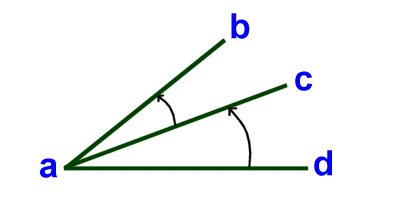Adjoining - When two objects share a common boundary, they are said to be adjoining.

Alternate exterior angles - When a third line called the transversal crosses two other (usually parallel) lines, angles are formed on the outside, or exterior, of the two lines. The angles that are opposite of each other are the alternate exterior angles.

In the picture below, a and b are alternate exterior angles as are c and d.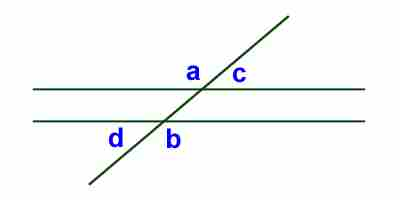Alternate interior angles - When a third line called the transversal crosses two other (usually parallel) lines, angles are formed on the inside, or interior, of the two lines. The angles that are opposite of each other are the alternate interior angles.

In the picture below, a and b are alternate interior angles as are c and d.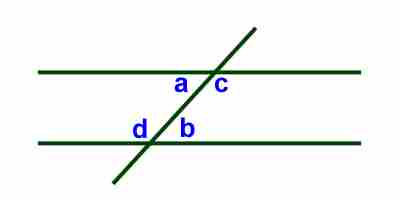Angle - An angle is a figure formed by two rays sharing a common endpoint called the vertex of the angle.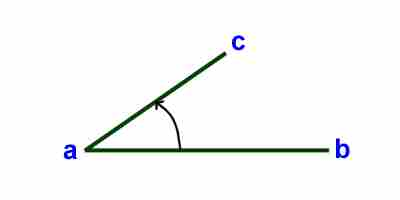Central angle - In a circle, a central angle has its vertex at the center of the circle and endpoints at the circumference.

Complementary angles - Two angles are complementary if they add up to 90°.

Corresponding angles - When two lines (usually parallel) are crossed by another (called the transversal) the angles in the same corners of each line are called corresponding angles.

In the picture below, a and d are corresponding angles as are c and b.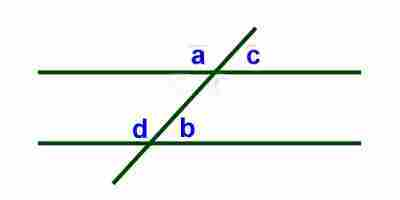Exterior angle - An exterior angle of a polygon is an angle between one side of a shape and a line that is extended from another side.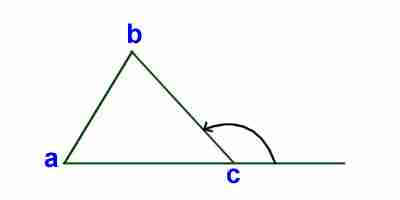Example of an exterior angle

Inscribed angle - An inscribed angle is an angle where the vertex as well as the end points all are on the circumference of a circle.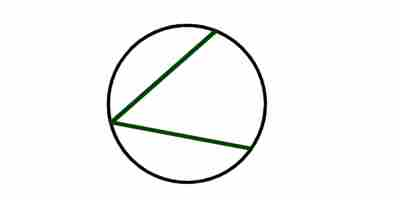Example of an inscribed angle

Obtuse angle - Any angle greater than 90°, but less than 180°.

Protractor - A protractor is a tool used for measuring angles and degrees.

Right angle - An angle that equals 90°.

Straight angle - An angle that equals 180°. It will look like a straight line.

Supplementary angles - When the sum of two angles is 180°, they are said to be supplementary.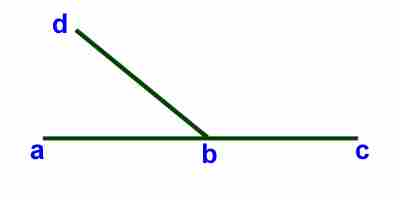Example of supplementary angles: ∠cbd and ∠dba are supplementary angles.

Transversal - A transversal is a line that crosses two or more other lines.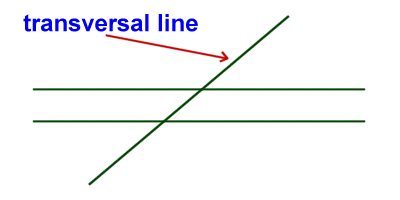More Math Glossaries and Terms

Algebra glossary
Angles glossary
Figures and Shapes glossary
Fractions glossary
Graphs and lines glossary
Measurements glossary
Mathematical operations glossary
Probability and statistics glossary
Types of numbers glossary
Units of measurements glossary

Back to Kids Math

Back to Kids StudyFollow us onor